Math Olympiad Test: Co-ordinate Geometry- 1

# Math Olympiad Test: Co-ordinate Geometry- 1 - Class 10

Test Description

## 10 Questions MCQ Test Olympiad Preparation for Class 10 - Math Olympiad Test: Co-ordinate Geometry- 1

Math Olympiad Test: Co-ordinate Geometry- 1 for Class 10 2023 is part of Olympiad Preparation for Class 10 preparation. The Math Olympiad Test: Co-ordinate Geometry- 1 questions and answers have been prepared according to the Class 10 exam syllabus.The Math Olympiad Test: Co-ordinate Geometry- 1 MCQs are made for Class 10 2023 Exam. Find important definitions, questions, notes, meanings, examples, exercises, MCQs and online tests for Math Olympiad Test: Co-ordinate Geometry- 1 below.
 1 Crore+ students have signed up on EduRev. Have you?
Math Olympiad Test: Co-ordinate Geometry- 1 - Question 1

### Find the value of k for which the points A (3, 2), B (4, k) and C (5, 3) are collinear.

Detailed Solution for Math Olympiad Test: Co-ordinate Geometry- 1 - Question 1

The points A (3, 2), B (4, K), and C (5, 3) are collinear then area (ΔABC) = 0
⇒ 1/2 [3(k − 3)+ 4(3 − 2) + 5(2 − k)] = 0
⇒ 3k - 9 + 4 + 10 - 5k = 0
⇒ -2k + 5 = 0 ⇒ k = 5/2

Math Olympiad Test: Co-ordinate Geometry- 1 - Question 2

### Find ratio in which the line 2x + y - 4 = 0 divides the line segment joining A(2, -2) and B(3, 7).

Detailed Solution for Math Olympiad Test: Co-ordinate Geometry- 1 - Question 2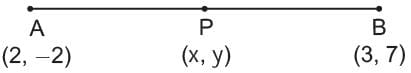Let the point P (x, y) divide the line AB in the ratio k : 1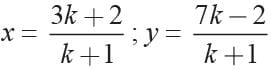This point P(x, y) lies on the line
2x + y - 4 = 0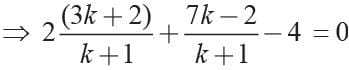⇒ 6k + 4+ 7k - 2 - 4k - 4 = 0
⇒ 9k - 2 = 0 ⇒ 9k = 2
⇒ k = 2/9 = 2 : 9

Math Olympiad Test: Co-ordinate Geometry- 1 - Question 3

### The co-ordinates of ends of a diameter of a circle are (4, -1) and (-2, -5). Find the centre of the circle.

Detailed Solution for Math Olympiad Test: Co-ordinate Geometry- 1 - Question 3

Ends of diameter of circle are (4, -1) and (-2, -5)
centre = Mid-point of (4, -1) and (-2, -5)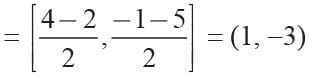Math Olympiad Test: Co-ordinate Geometry- 1 - Question 4

The area of a triangle with vertices (a, b + c) and (b, c + a) and (c, b + a) is

Detailed Solution for Math Olympiad Test: Co-ordinate Geometry- 1 - Question 4

Area of triangle
= 1/2 [a(c + a - a - b) + b (a + b - b - c) + c (b + c - c - a)]
= 1/2 [a(c − b)+ b(a − c) + c(b − a)]
= 1/2 [ac − ab + ab − bc + bc − ac]
= 1/2 × 0 = 0

Math Olympiad Test: Co-ordinate Geometry- 1 - Question 5

Find the co-ordinates of the points which trisects the line joining (-3, 5) and (6, -7).

Detailed Solution for Math Olympiad Test: Co-ordinate Geometry- 1 - Question 5

Let P(x, y) be the point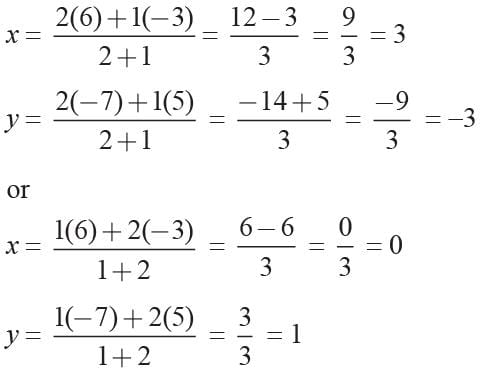Math Olympiad Test: Co-ordinate Geometry- 1 - Question 6

The ends of a diagonal of a square have the coordinates (a, 1) and (-1, a), find a if the area of the square is 50 square units.

Detailed Solution for Math Olympiad Test: Co-ordinate Geometry- 1 - Question 6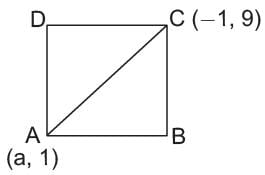Let side of the square be x.
x2 + x2 = (-1 - a)2 + (a - 1)2
⇒ 2x2 = 1 + a2 + 2a + a2 + 1 - 2a
⇒ x2 = a2 + 1
⇒ 50 = a2 + 1 ⇒ a2 = 49
⇒ a = ±7

Math Olympiad Test: Co-ordinate Geometry- 1 - Question 7

Two vertices of a triangle are (2, -4) and (1, 3). If the origin is the centroid of the triangle then what is the third vertex?

Detailed Solution for Math Olympiad Test: Co-ordinate Geometry- 1 - Question 7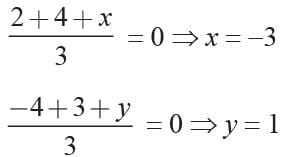third vertex = (-3, 1)

Math Olympiad Test: Co-ordinate Geometry- 1 - Question 8

What is the locus of a point equidistant from the point (2, 4) and y-axis?

Detailed Solution for Math Olympiad Test: Co-ordinate Geometry- 1 - Question 8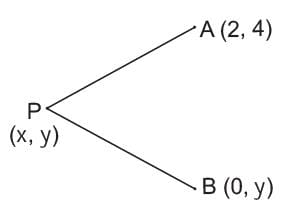Let, the point be P(x, y).
Given AP = BP
⇒ AP2 = BP2
⇒ (x - 2)2 + (y - 4)2 = (x - 0)2 + (y - y)2
⇒ x2 + 4 - 4x + y2 + 16 - 8y = x2
⇒ y2 - 4x - 8y + 20 = 0

Math Olympiad Test: Co-ordinate Geometry- 1 - Question 9

If the coordinates of the mid-point of the sides of a triangle are (1, 1) (2, -3), and (3, 4) what is the centroid?

Detailed Solution for Math Olympiad Test: Co-ordinate Geometry- 1 - Question 9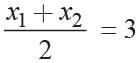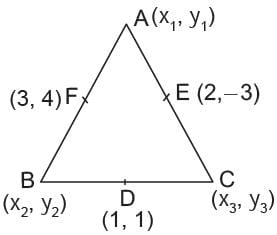⇒ x1 + x2 = 6
y1 + y2 = 8
x2 + x3 = 2
y2 + y3 = 2
x1 + x3 = 4
y1 + y3 = -6
x1 + x2 + x3 = 12/2 = 6
x1 = 6 - 2 = 4; x2 = 2, x3 = 0
y1 + y2 + y3 = 2
y1 = 0; y2 = 8; y3 = -6
Centroid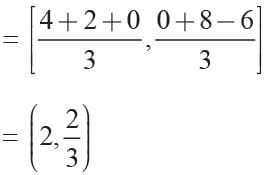Math Olympiad Test: Co-ordinate Geometry- 1 - Question 10

What is the value of k, so that the points A(8, 1), B(3, -4), and C(2, K) are collinear?

Detailed Solution for Math Olympiad Test: Co-ordinate Geometry- 1 - Question 10

Given points are A(8, 1), B(3, −4) and C(2, k)
It is also said that they are collinear and hence the area enclosed by them should be 0
Area of the triangle having vertices (x1, y1), (x2, y2) and (x3, y3
= 1/2 |x1(y- y3) + x2(y- y1) + x3(y- y2)|
Given that area of ∆ABC = 0
∴ 0 = 1/2 |8(-4 – k) + 3(k – 1) + 2(1 – (-4))|
∴ 0 = 1/2 |-32 – 8k + 3k - 3 + 10|
∴ 5k + 25 = 0
∴ k = -5
Hence, the value of k is -5.

## Olympiad Preparation for Class 10

11 videos|36 docs|201 tests